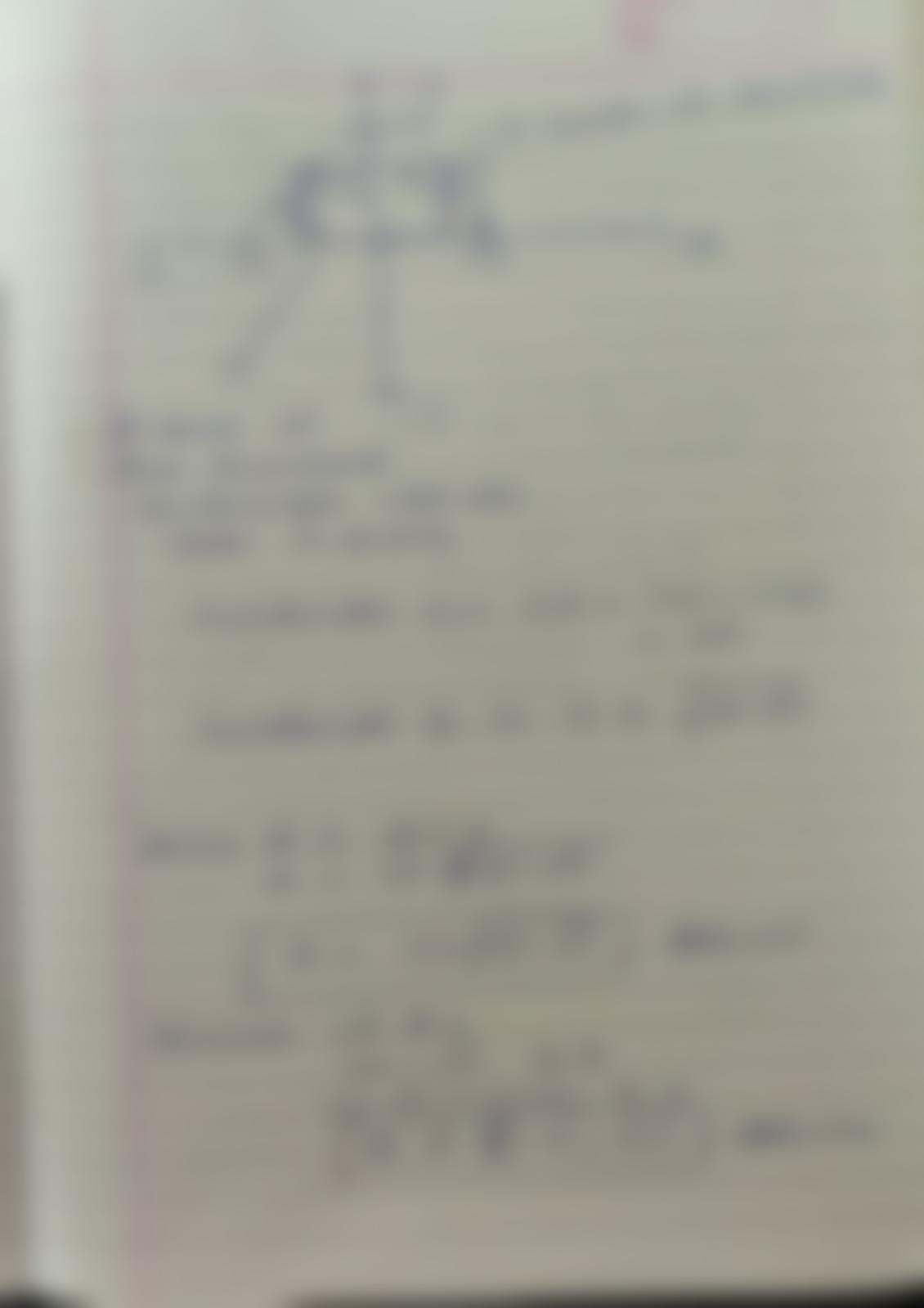Question:

uuuuule WIFUUW LU see the a. whole graph. d. Find the maximum Volume (vertex) this box can have. 7. CHALLENGE GEOMETRY APPLICATIuuuuule WIFUUW LU see the a. whole graph. d. Find the maximum Volume (vertex) this box can have. 7. CHALLENGE GEOMETRY APPLICATION: A rectangle is bounded by the x-axis and the semicircle y= V36 - 42 Write the Area of the rectangle as a function of x and determine the domain of the function. Hint: Make a T table. b. Draw a picture of the semicircle. Determine the Domain (possible x values for this equation) d. Draw the rectangle inscribed inside of it. e. Use the graph to determine points where the corners of the rectangle touch the semicircle. f. Area is Length *Width. A=lw g. The length is 2x in your diagram. h. The width is y = V36 - x2 C. - of Functions T-